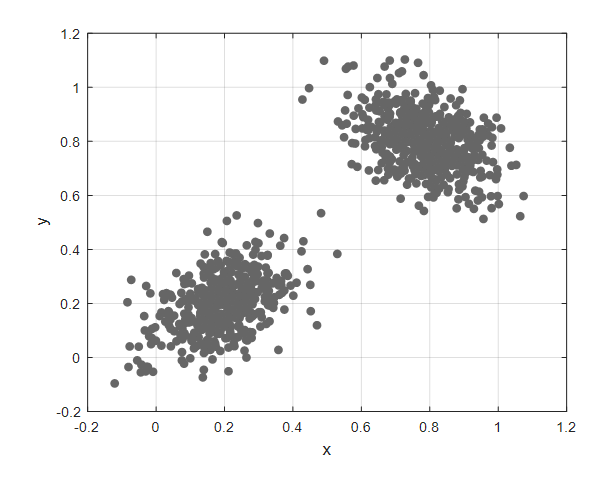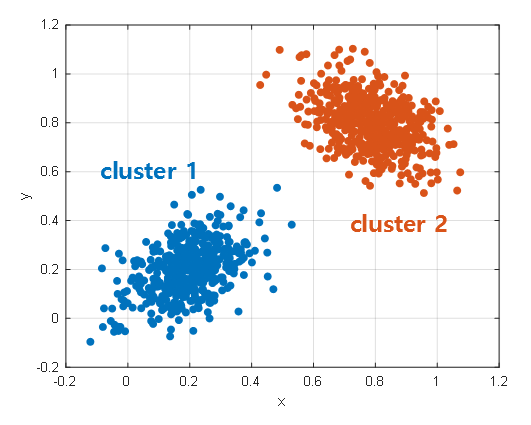# Clustering problem

Machine learning can be broadly divided into supervised learning problems and unsupervised learning problems. One of the most commonly used applications in unsupervised learning is clustering.

Clustering is the process of labeling groups of closely located data for a given set of training data $\lbrace x^{(1)},\cdots,x^{(m)}\rbrace$.

Suppose the data is given as in Figure 1. At this time, let’s assume that each data point is not labeled.Figure 1. Dataset without labels. How should this dataset be grouped into two clusters?Figure 2.

Figure 3. Process of performing k-means algorithm

# k-means Algorithm

Initialize cluster centroids $\mu_1, \mu_2, \cdots, \mu_k \in \Bbb{R}^n$ randomly

Repeat Until Convergence: {

$\quad$ For every $i$, set

$c^{(i)}=\underset{j}{\text{arg min}} ||x^{(i)}-\mu_j||^2$

$\quad$ For every $j$, set

$\mu_j := \frac{\sum_{i=1}^{m}1\lbrace c^{(i)}=j\rbrace x^{(i)}}{\sum_{i=1}^{m}1\lbrace c^{(i)}=j\rbrace}$

}

Here, $k$ is the number of clusters (hence, k-means algorithm). There are several ways to help choose the number of clusters, but ultimately, it is inevitable to visually inspect the data and decide on the value of $k$.

Also, the function $1\lbrace \text{condition} \rbrace$ outputs 1 if the condition is True, and 0 otherwise. That is, the function $1\lbrace c^{(i)}=j\rbrace$ outputs 1 if the class of the $i$-th data is $j$, and 0 otherwise.